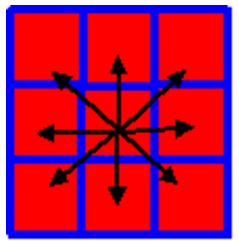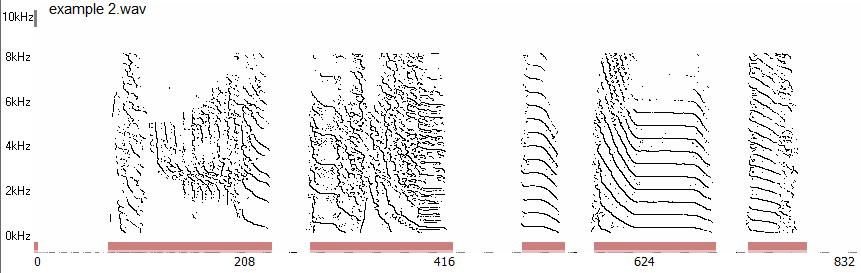# Frequency Contours

 < PREVIOUS: Segmentation of Syllable Units > NEXT: Chapter 4, song features SAP2011 calculates frequency contours by detecting zero crossings of the spectral derivatives. In order to reject artifact, we require that the contours passed a dynamic contrast threshold, T, calculated for each time window ti and frequency fi as: T(ti,fi)=abs(Wiener_entropy(ti)) / abs(fi-mean_frequency(ti)), were T’ is a user defined threshold. Therefore, the detection threshold is weighted by the distance from the mean frequency (the gravity center of the frequencies) and by the width of the power spectrum. A pixel in the time frequency space is defined as contour if i) there is zero crossing between the neighboring pixels at any one of the 8 possible directions (see diagram below) and ii) both neighboring pixels (in the direction of the zero crossing) are larger than T.Here is an example with T'=10:and with T'=50: